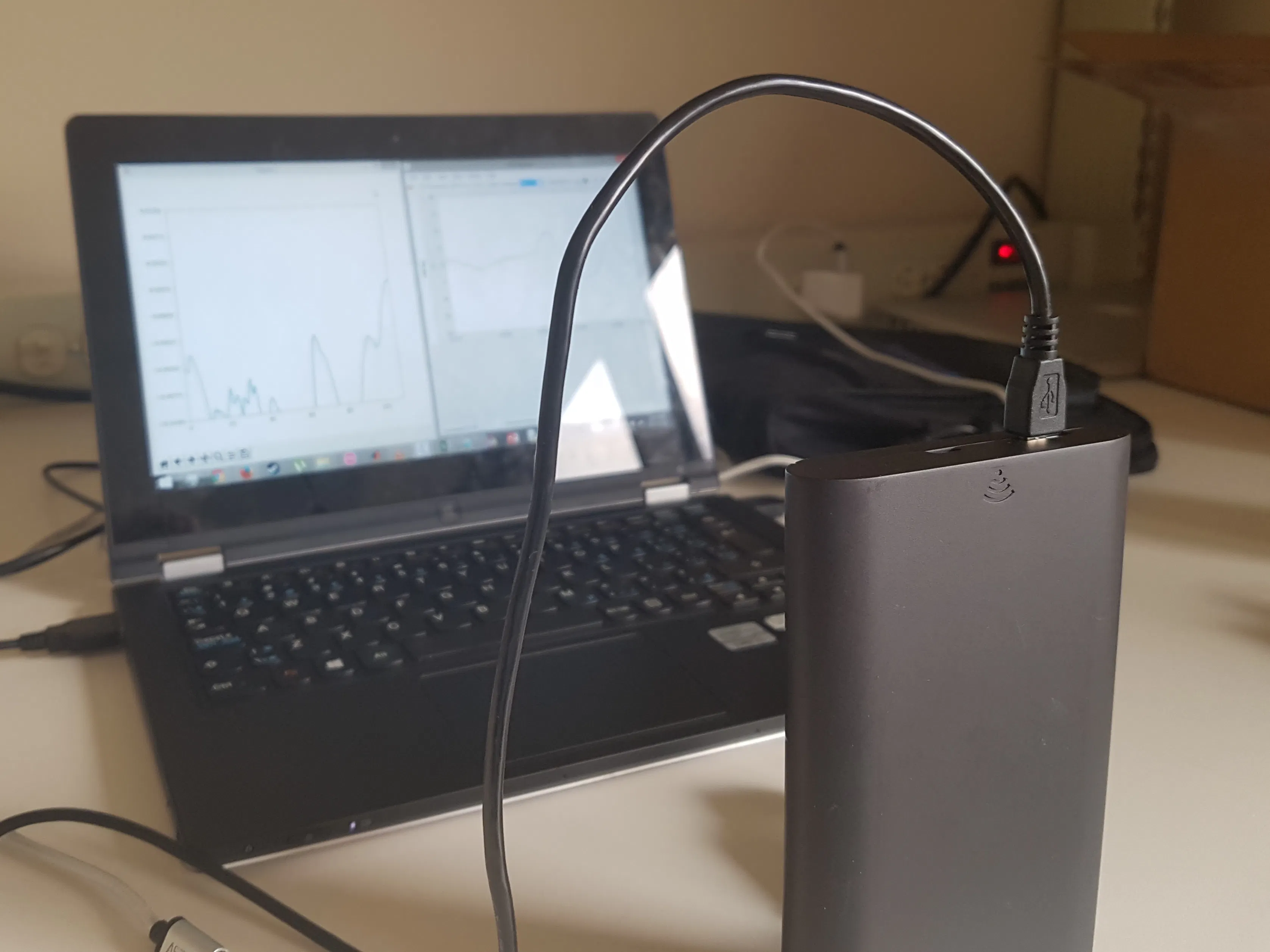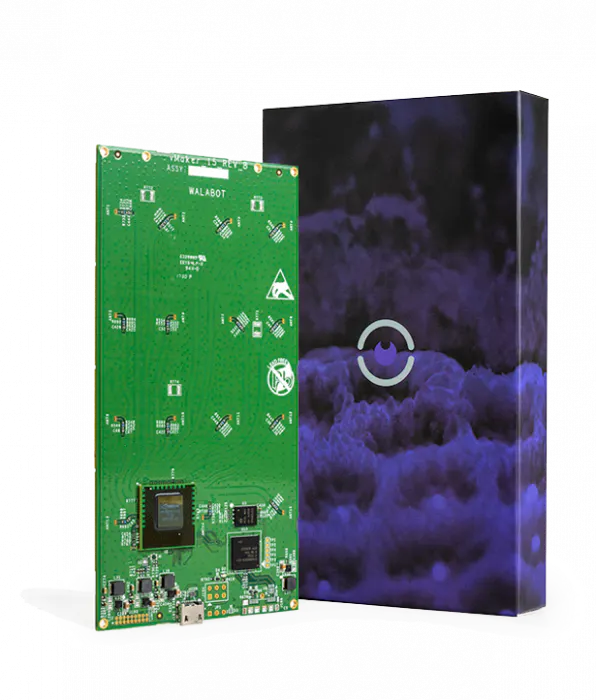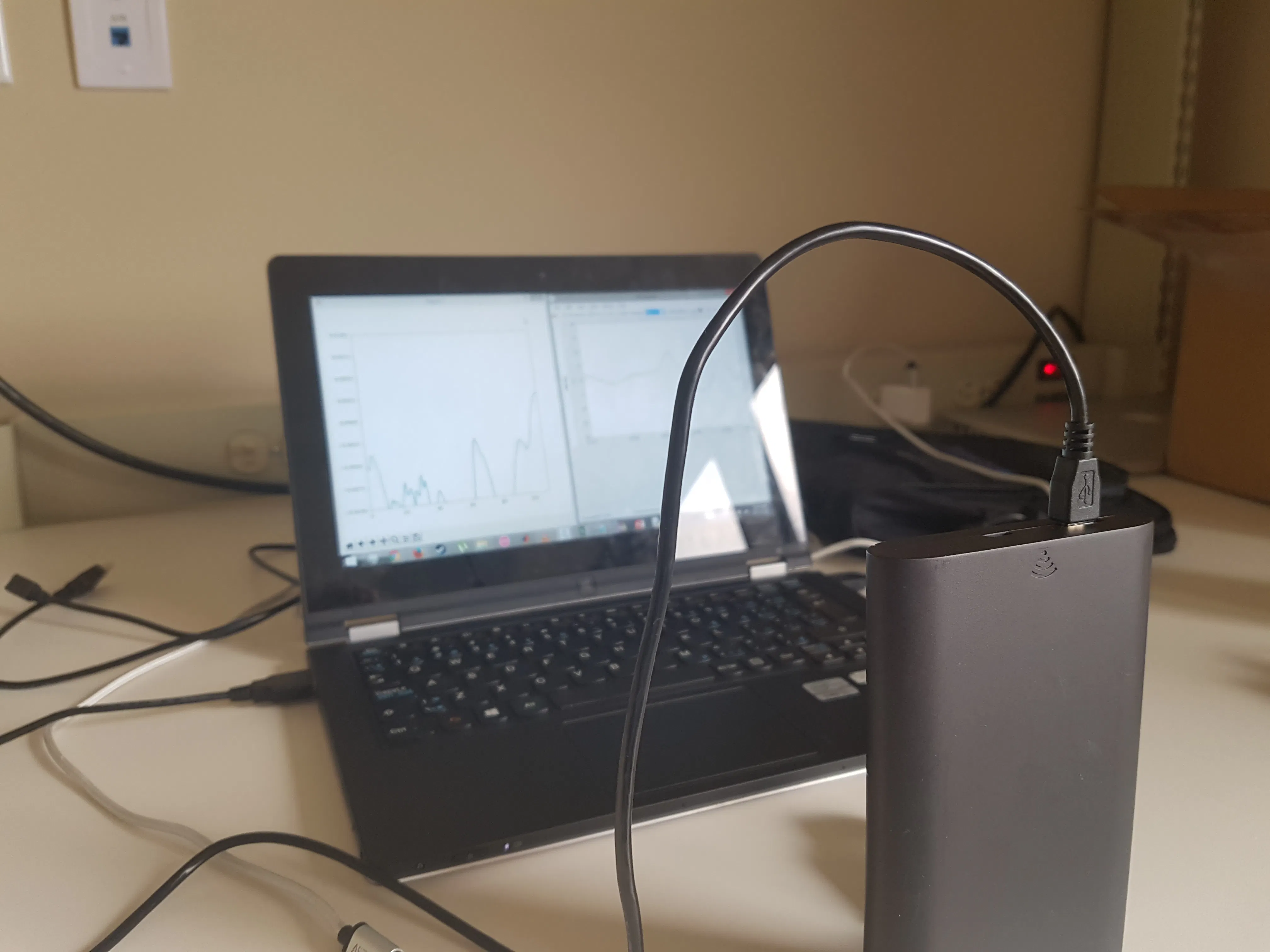Published

# Testing the Viability of Walabot for Breathing Detection

By comparing the Walabot as a breathing detector to a known gold standard, it's viability as a wireless breathing detector can be shown.

BeginnerWork in progress1,345## Things used in this project

### Hardware components

×1Walabot Creator
×1

## Schematics

### Walabot Connected to PC with Microusb Cable

This allows the PC to control the Walabot and receive data from it.## Code

### Walabot Breathing Code

Python
This code is used to control Walabot as a breathing detector. It also writes this data to a file. This data is then averaged with the last 5 values, and then plotted in real time as the Walabot runs.
```import WalabotAPI as wlbt
import numpy as np
import pylab as plt
import time
from matplotlib import animation
from datetime import datetime

plt.ion()
wlbt.Init()  # load the WalabotSDK to the Python wrapper
wlbt.SetSettingsFolder()  # set the path to the essetial database files
wlbt.ConnectAny()  # establishes communication with the Walabot

wlbt.SetProfile(wlbt.PROF_SENSOR_NARROW)  # set scan profile out of the possibilities

R_MIN, R_MAX, R_RES = 20, 80, 0.2  # SetArenaR values
THETA_MIN, THETA_MAX, THETA_RES = -1, 1, 1  # SetArenaTheta values
PHI_MIN, PHI_MAX, PHI_RES = -1, 1, 1  # SetArenaPhi values

wlbt.SetDynamicImageFilter(wlbt.FILTER_TYPE_NONE)  # use no filter, which will find distance from walabot

wlbt.Start()  # starts Walabot in preparation for scanning

num = 110 # number of points to plot
# each point is about 0.0932 secconds in v2+
# each point is about 0.0929 secconds in v4+

ylimits = 0.01 # below 0.0005 is background for derivative mode
# 0.01 for NONE filter mode works for about 50cm

fig = plt.figure() # initialize plot
ax = plt.axes(xlim=(0,num), ylim=(-ylimits,ylimits))
line, = ax.plot([],[],lw=2)

y=np.zeros(num) # initalize y values
y_sm = np.zeros(num) # initalize smoothed y values

f = open('02_13-01.csv','w') # open file to write data to

# initialize plot at each frame
def init():
line.set_data([],[])
return line,

def animate(i):
x = np.linspace(1,num,num) # set up x values for plot
wlbt.Trigger() # trigger walabot
ener = wlbt.GetImageEnergy() # use image energy of walabot to detect breathing

# array of actual energy values
# stores the array of energy values to be used in averaging
global y
y = np.asarray(y)
y = np.roll(y,-1)
y = y.tolist() # shift the y values down by one
y[num-1] = ener

# array of averaged values
global y_sm
y_sm = np.asarray(y_sm)
y_sm = np.roll(y_sm,-1)
y_sm = y_sm.tolist() # shift the averaged y values down by one row
en_smth = np.sum(np.insert(y[num-5:num],0,0)) / 5 # averages the current y value with the previous 5 values
y_sm[num-1] = en_smth

# write to data
line.set_data(x,y_sm) # write new y value averages to plot
f.write(str(datetime.now())+ ',' + str(ener)+'\n') # write every value to a new line, with a timestamp

return line,

anim = animation.FuncAnimation(fig, animate, init_func=init,interval=1,blit=True)
plt.show()
```

## Credits

### Ramsey Kilani

1 project • 0 followers